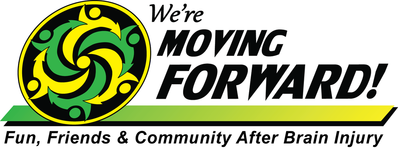# January 2020

 /* styles */ We kicked off the new year watching the Arizona Coyotes play against the Philadelphia Flyers for our first activity of 2020. Our seat location was great and the Coyotes won!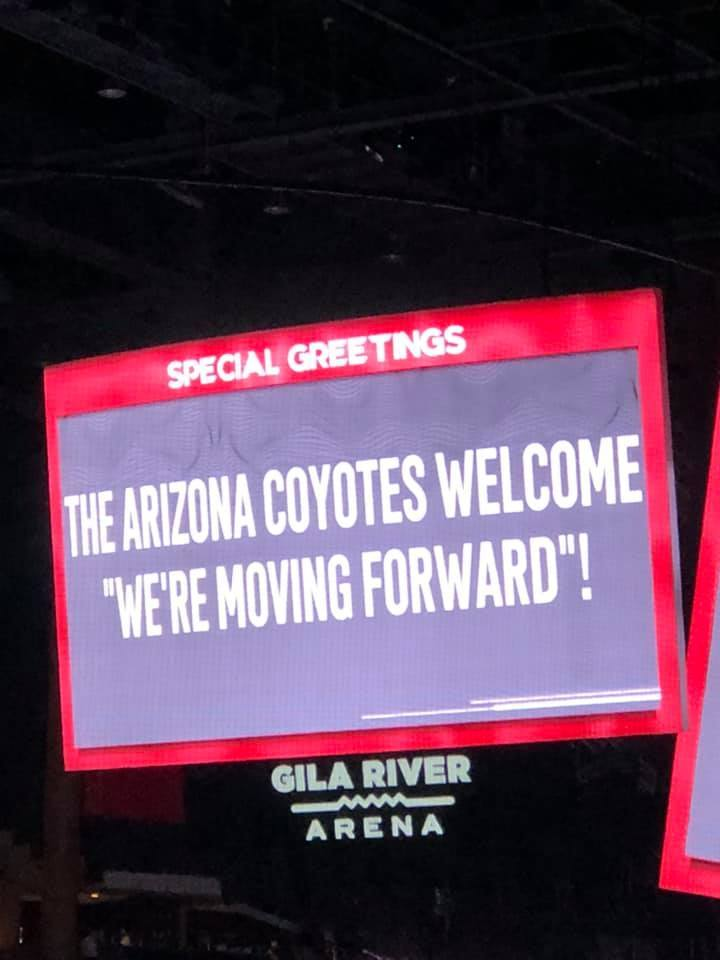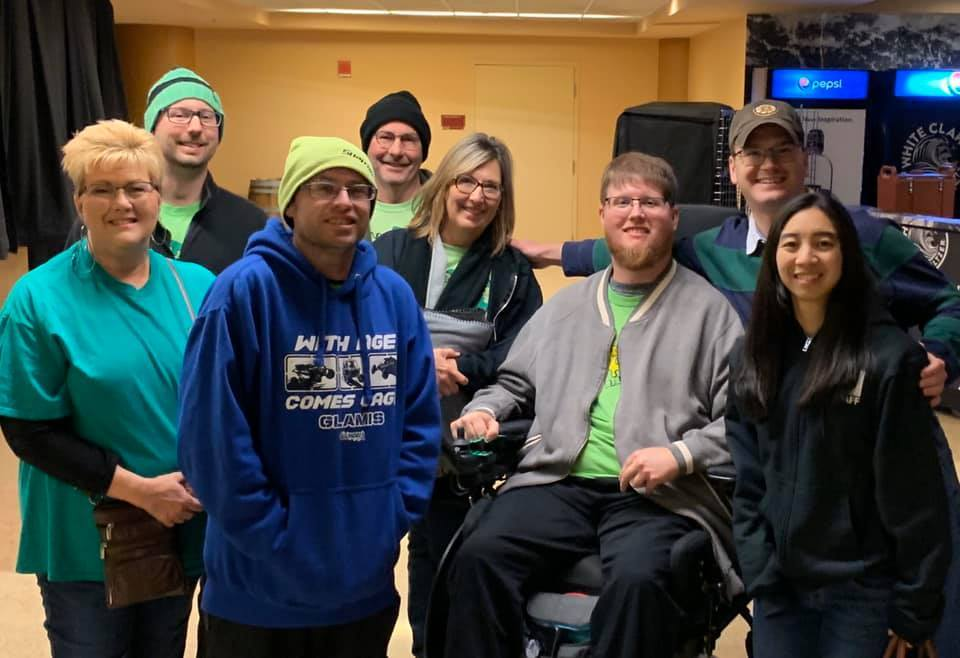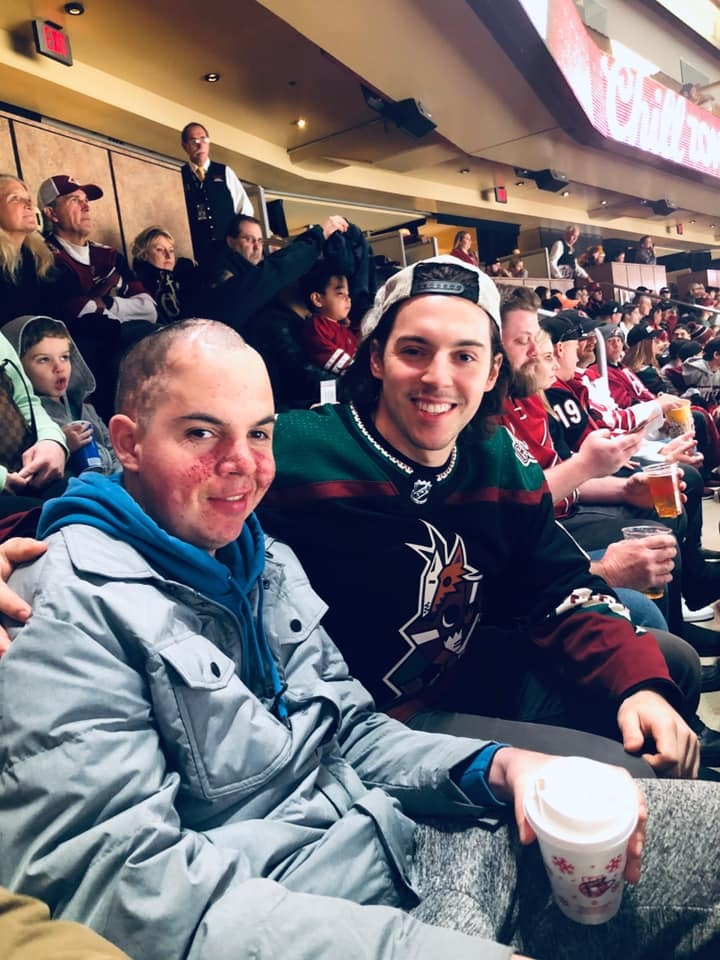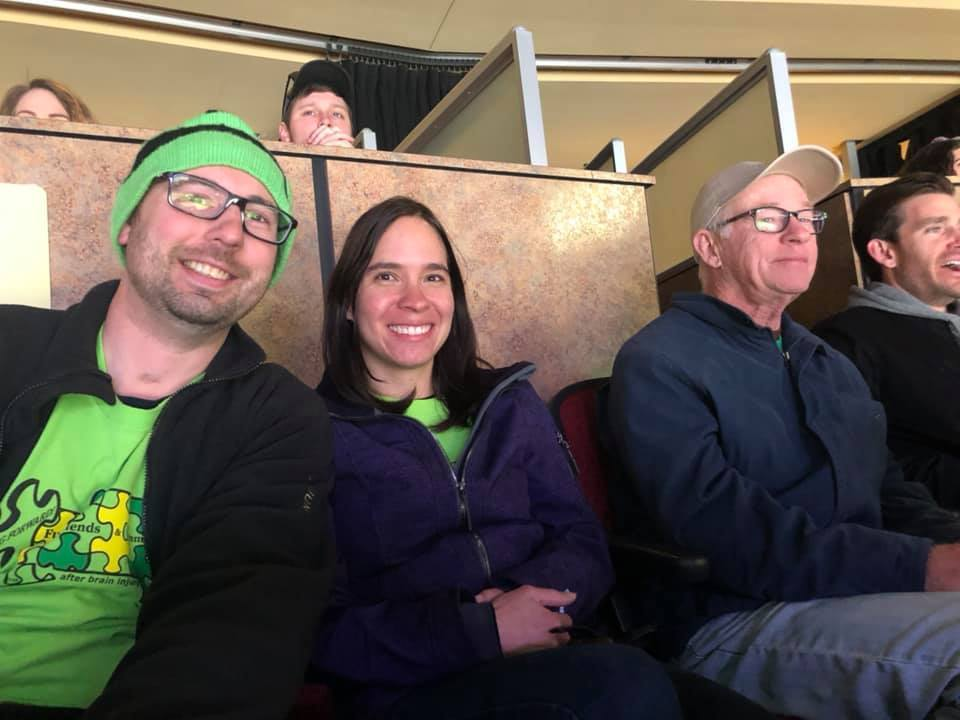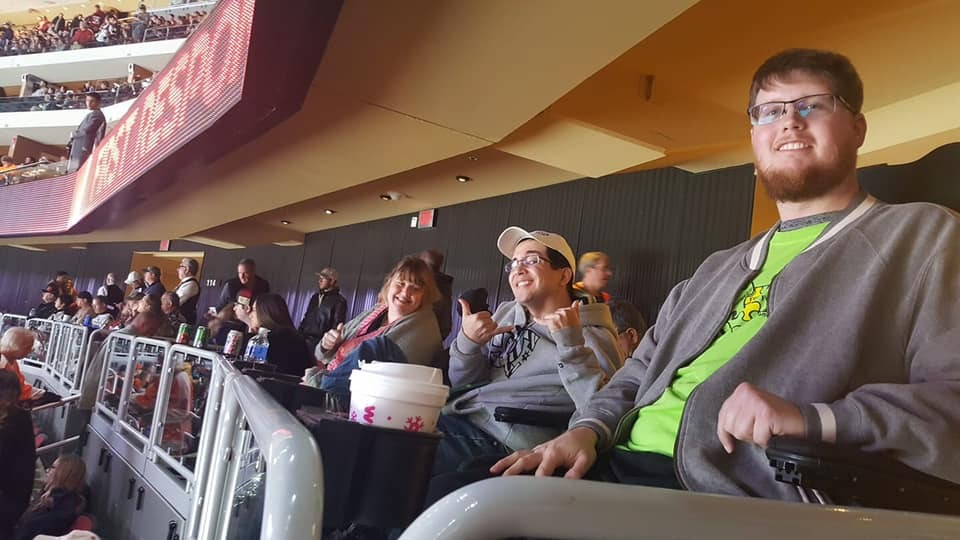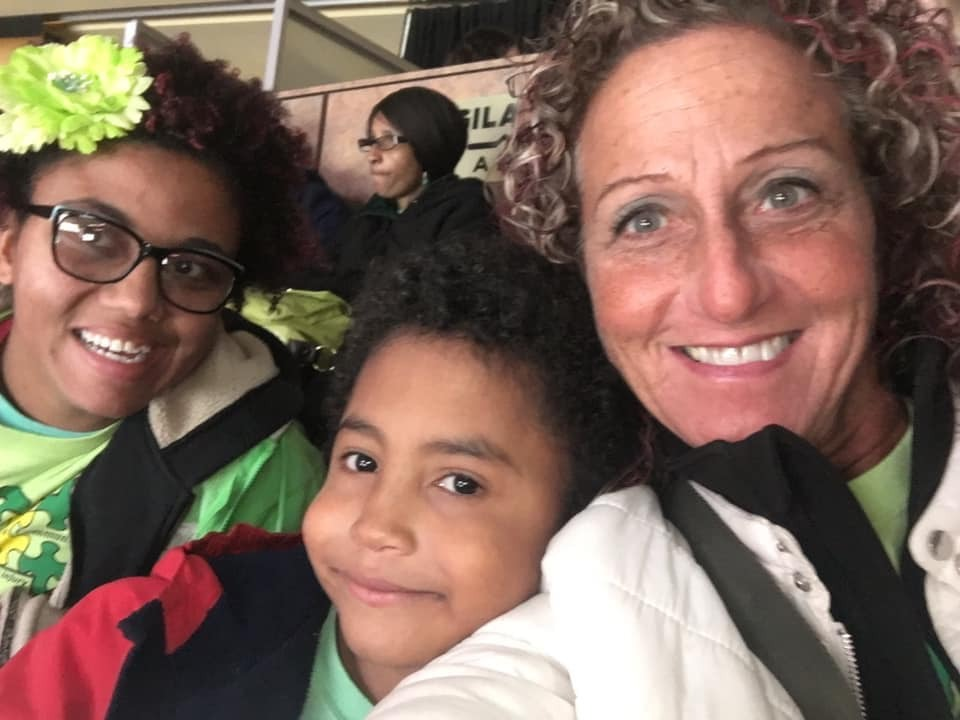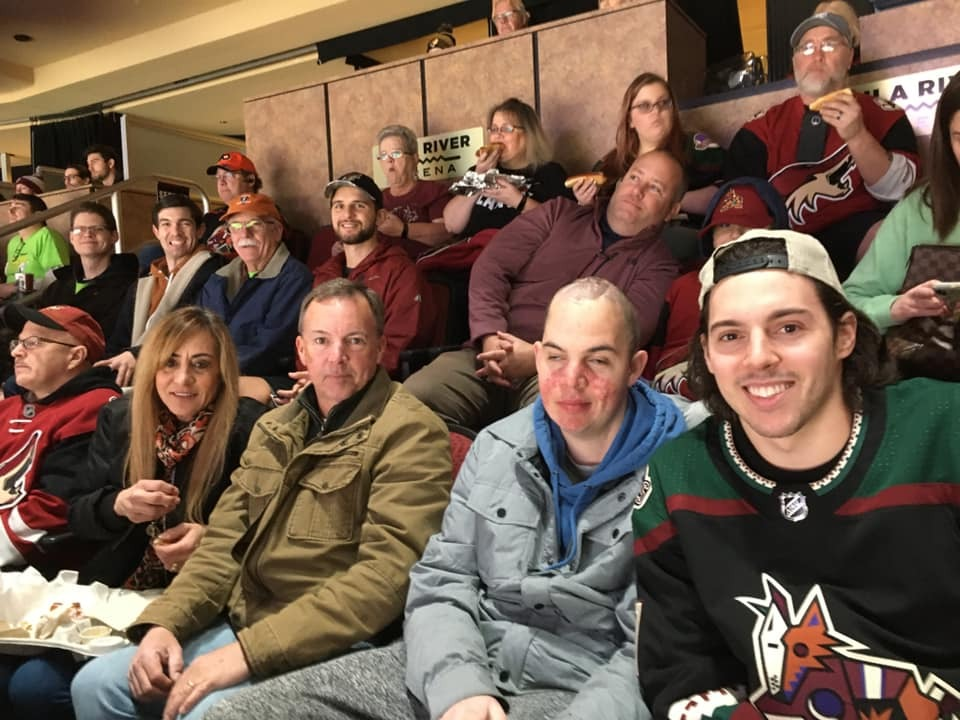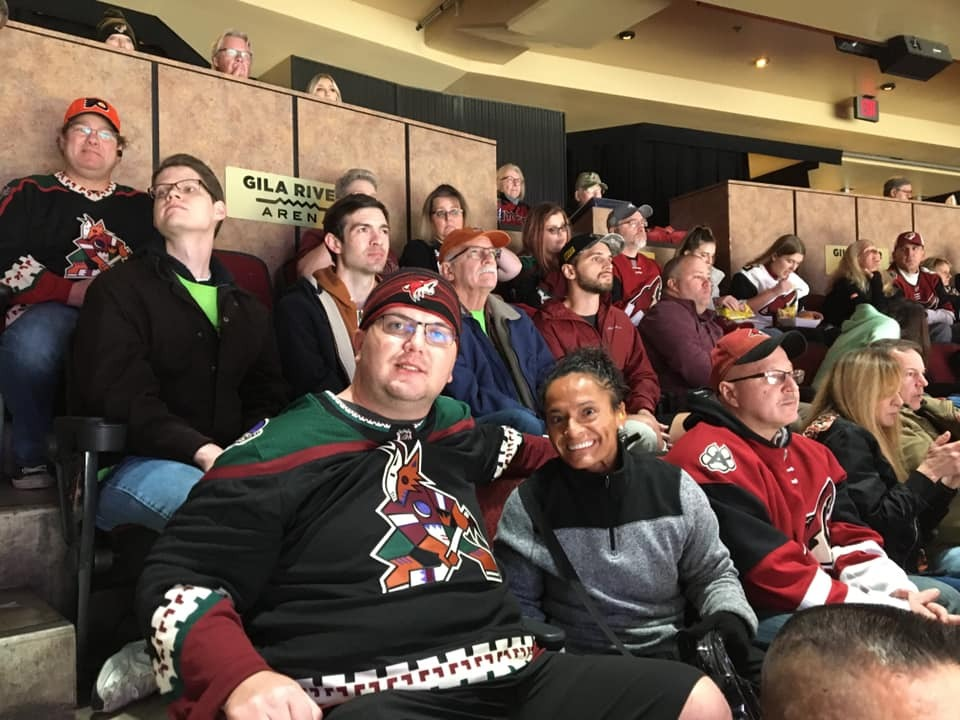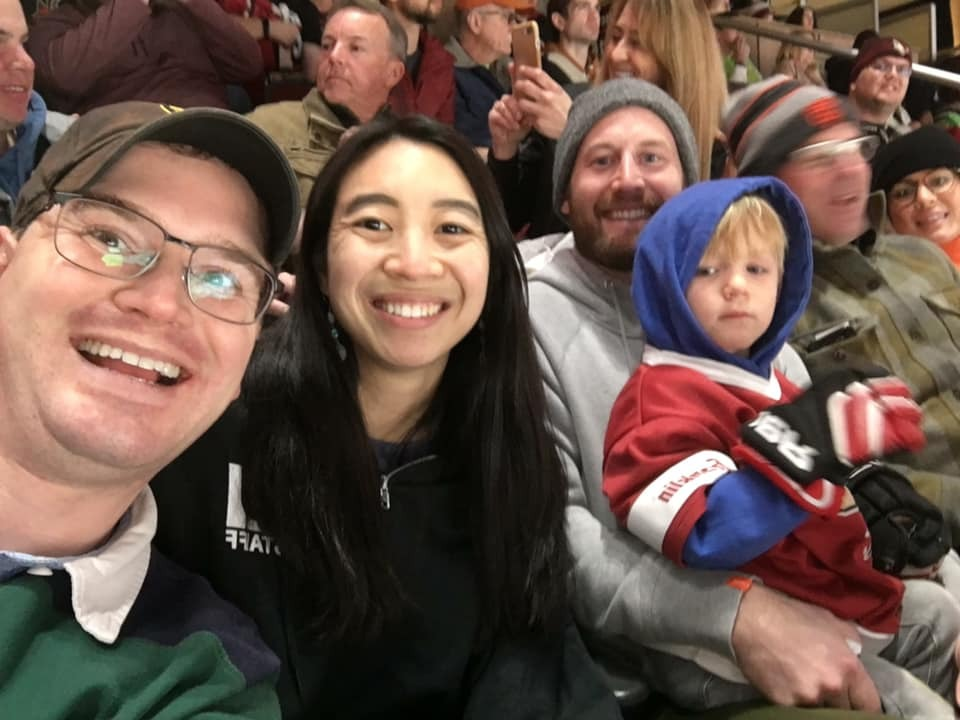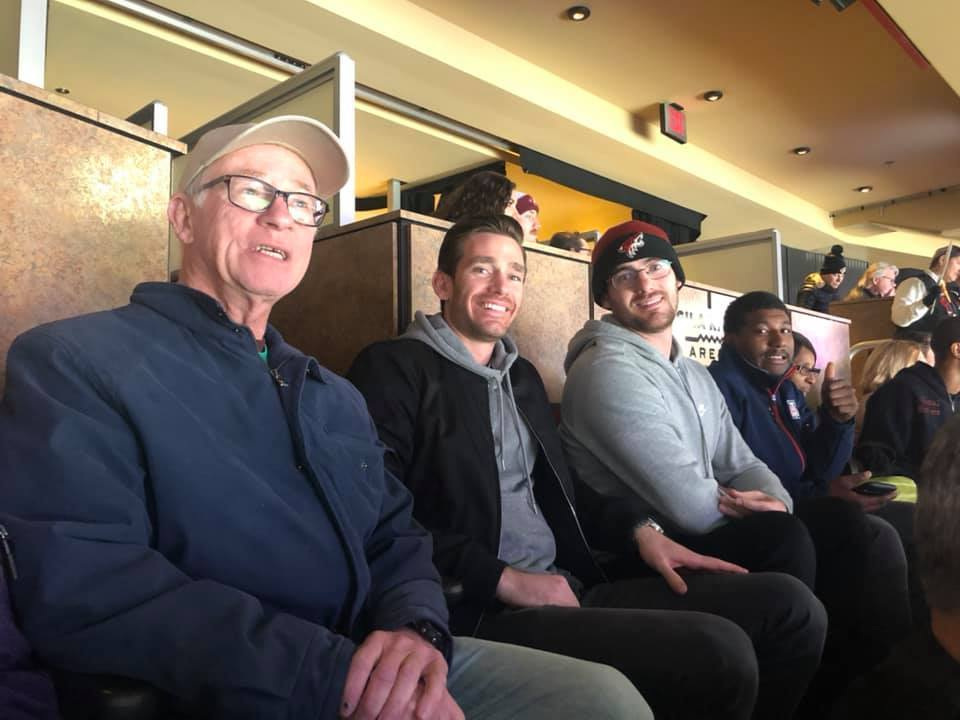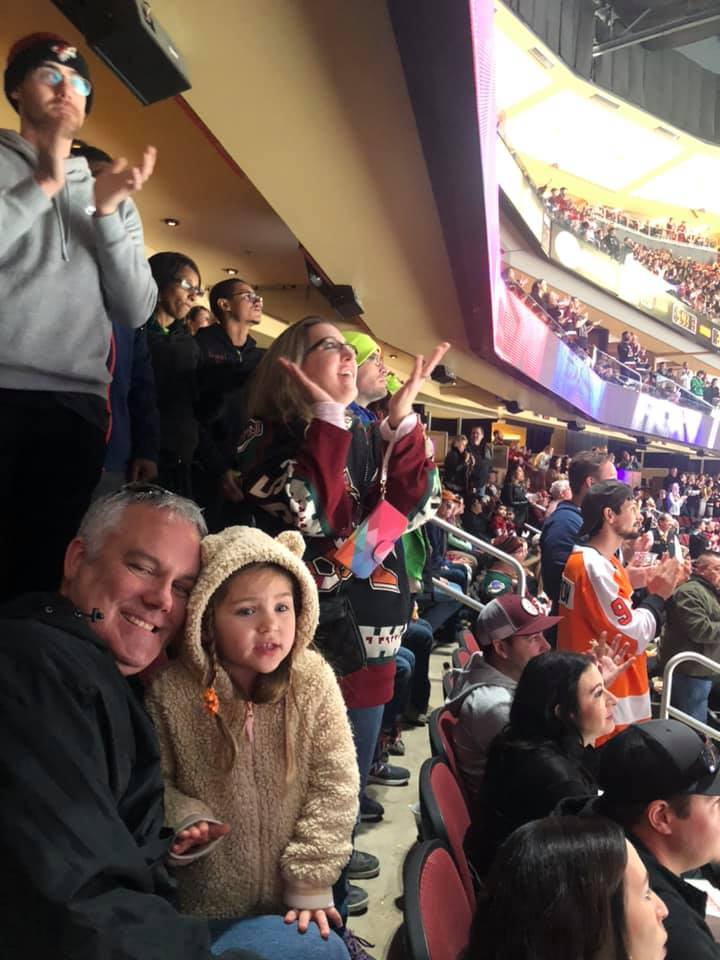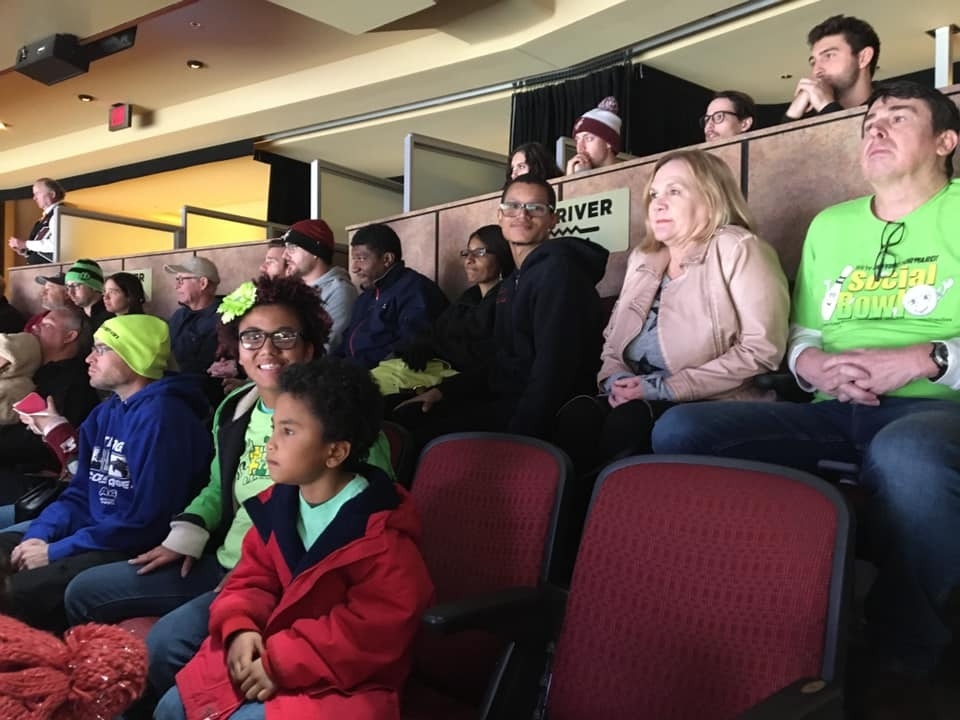table div table+table+table+table+table+table+table+table+table+table+table div table{width:100%;padding:0}table div table+table+table+table+table+table+table+table+table+table+table div table img{width:96.23%;padding:0;float:none}table div table+table+table+table+table+table+table+table+table+table+table div table td{width:100%;padding:0 1.88% 18px}/* styles */# New WMF Website

 /* styles */ Our new website is up and running! Take a look and let us know what you think. We are in the testing phase, therefore if you encounter any problems, please let us know at: info@wmforward.org
 /* styles */ The Buhr's are our first featured volunteers, and Jen Ayers is our first featured survivor. Click here to check them out!

## Feature Volunteers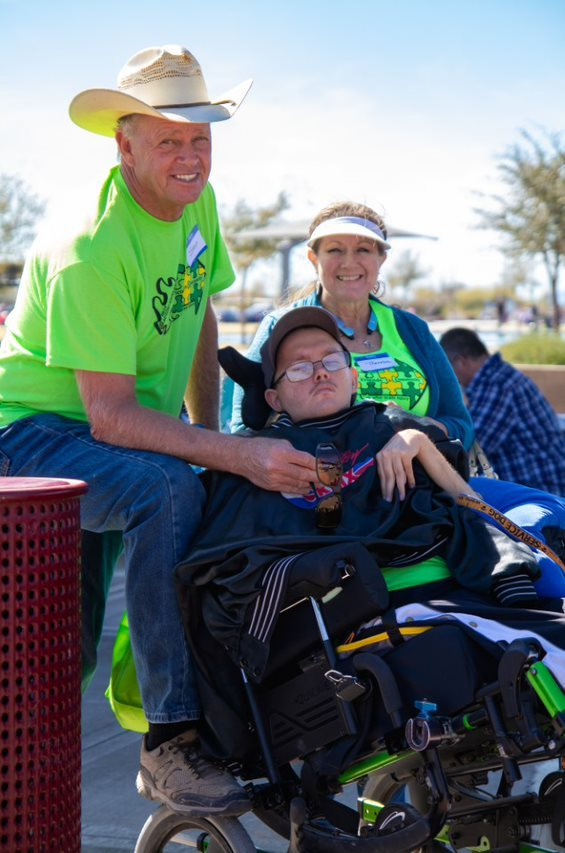## Feature Survivor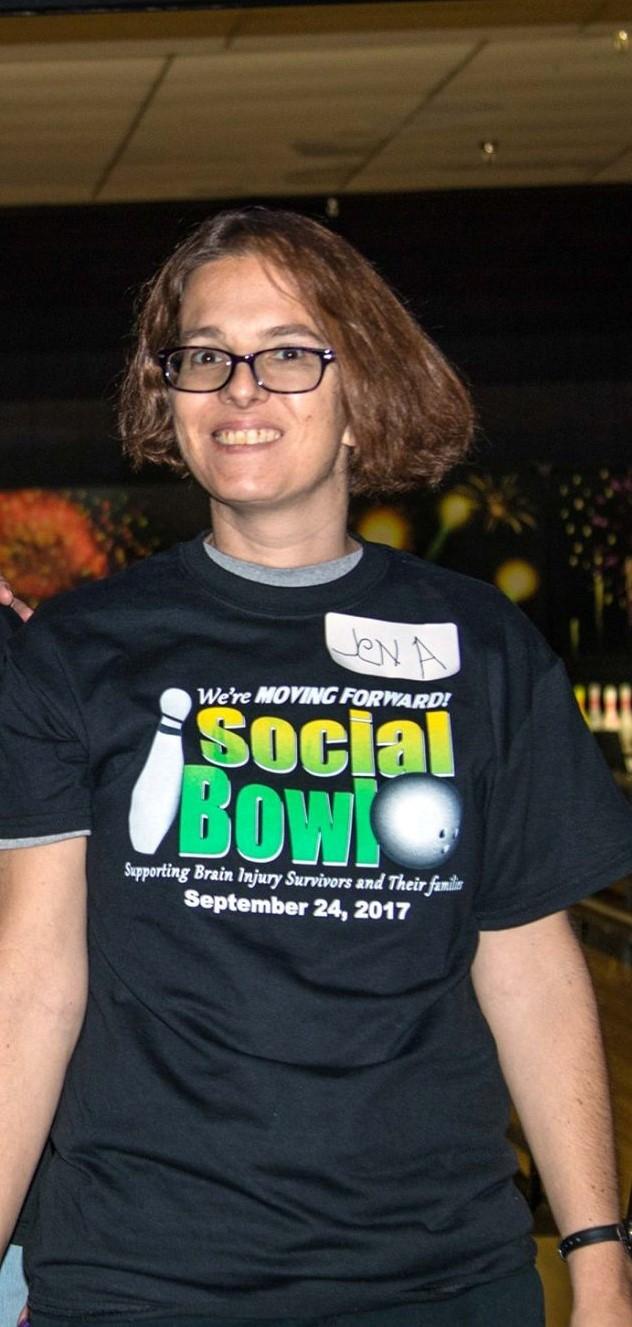# 2019 Year in Review Video

 /* styles */ To view our "2019 Year in Review" video, please visit our YouTube channel. While you are there, please make sure to subscribe to our channel and give us a thumbs up. We are grateful to Jason Stahl and the students at Eastmark High School for their time and talent in producing our video this year.
 table div table+table+table+table+table+table+table+table+table+table+table+table+table+table+table+table+table+table+table+table+table div table td,table.module-20{width:100%;padding:0}table div table+table+table+table+table+table+table+table+table+table+table+table+table+table+table+table+table+table+table+table+table div table{width:100%;float:none;margin-left:auto;margin-right:auto;padding:0}table div table+table+table+table+table+table+table+table+table+table+table+table+table+table+table+table+table+table+table+table+table div table a{border:0 none;text-decoration:none}table div table+table+table+table+table+table+table+table+table+table+table+table+table+table+table+table+table+table+table+table+table div table img{width:100%!important;border:0 none;text-decoration:none}/* styles */

# Are you Registered?

Registration is currently open for almost all of our 2020 activities and events.

The majority of the activities and events are FREE for survivors and their immediate family/caregivers. Everyone else is welcome to join us at our discounted costs.

 /* styles */
 table div table+table+table+table+table+table+table+table+table+table+table+table+table+table+table+table+table+table+table+table+table+table+table+table div table{width:100%;padding:0}table div table+table+table+table+table+table+table+table+table+table+table+table+table+table+table+table+table+table+table+table+table+table+table+table div table img{width:96.23%;padding:0;float:none}table div table+table+table+table+table+table+table+table+table+table+table+table+table+table+table+table+table+table+table+table+table+table+table+table div table td{width:100%;padding:0 1.88% 18px}/* styles */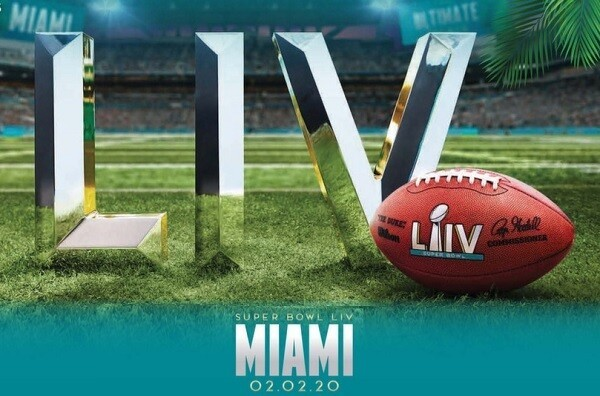February 2nd

Super Bowl Social

Top Golf
Gilbert

Ages 18+

FREE to survivors, immediate family/caregivers

\$24 everyone else

(Includes food)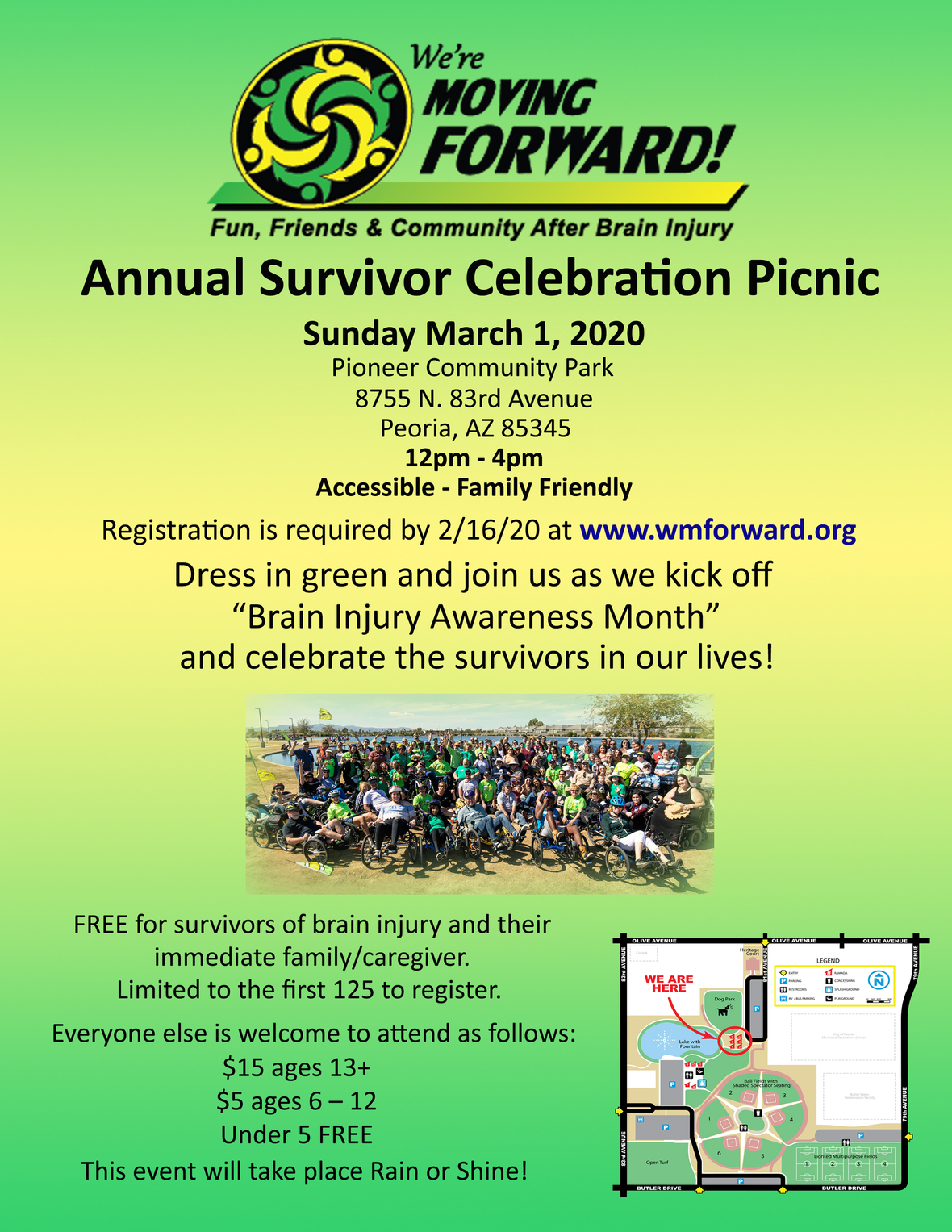March 15th

Everyone is welcome!

We are trying something new this year!

Instead of a golf course, we will be at Top Golf in Gilbert!

Stay tuned for participant registration and sponsorship opportunities.March 18th

Cucina Tagliani

Glendale

Everyone is welcome!

Dinner is your own cost, as this is also a fundraiser for WMF.

Make sure to sign up to let us know you are attending.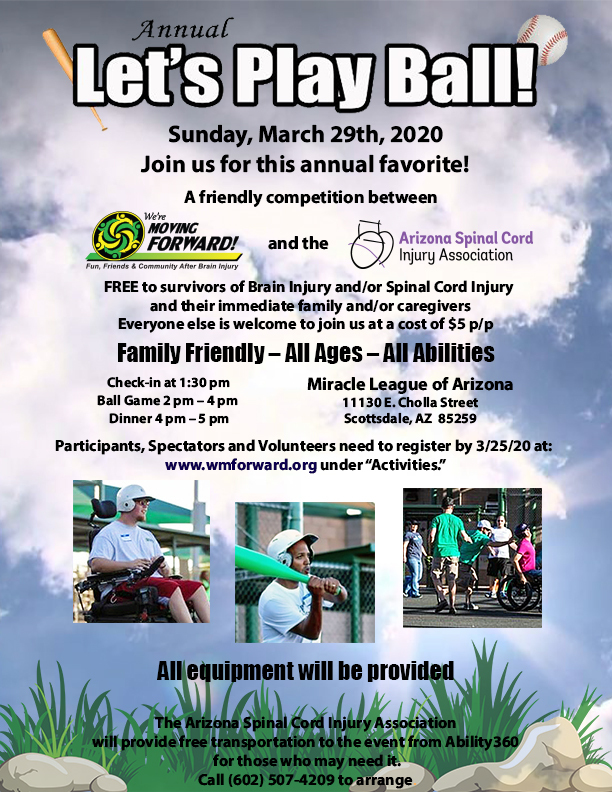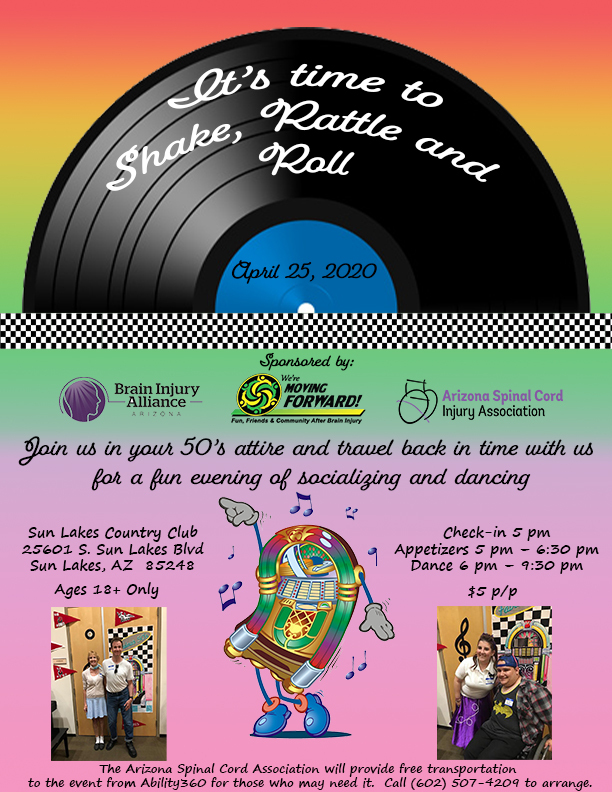May 23rd

Ability360

Phoenix

Ages 18+

FREE for survivors and immediate family/caregivers

\$5 Everyone Else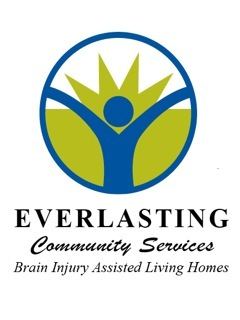We thank Everlasting Community Services for helping to sponsor our first ever Bingo Night!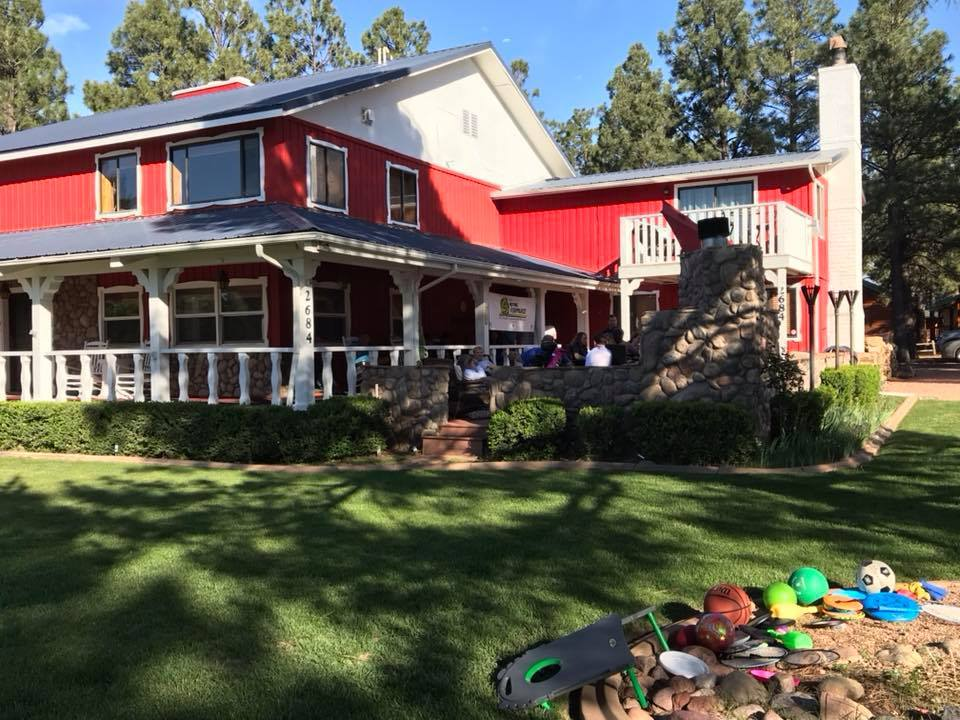June 12th - June 14th

Overgaard

Everyone is welcome AND we need volunteers!

There is currently 1 upstairs room with a queen bed and 1 upstairs room with 2 twin beds available at the All Seasons Lodge. First to make a deposit to reserve gets them!

Everyone else needs to make their own reservations within / near Bison Ranch in Overgaard (not Show Low)

Visit our website for details and suggestions of places to stay. It is recommended you make your reservations as soon as possible.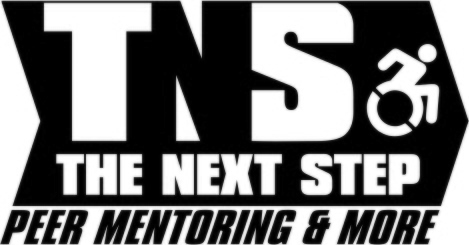We thank the Next Step Peer Mentoring & More organization for providing kayaking opportunities for our participants at Willow Springs Lake on 6/13/20.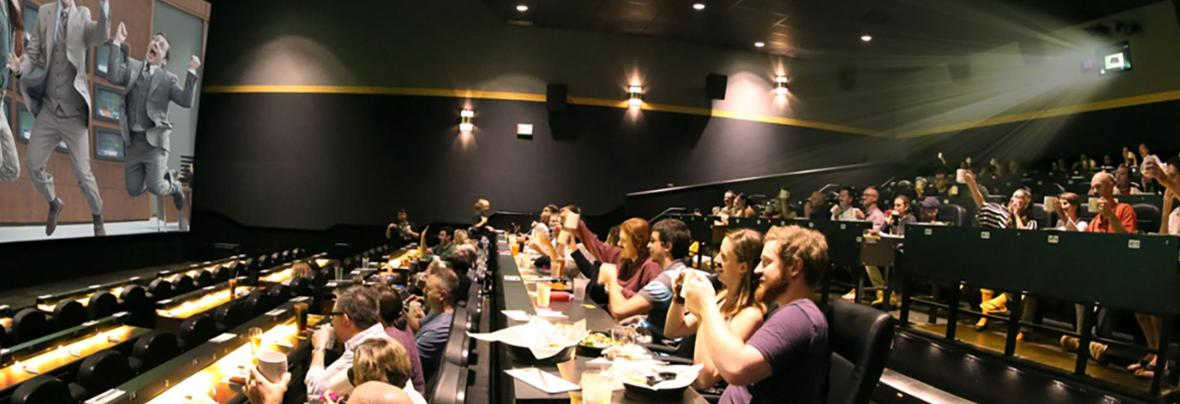July 18th

Flix Brewhouse (Movie TBD)

Chandler

Ages 18+

FREE for survivors and immediate family/caregivers

\$16 Everyone else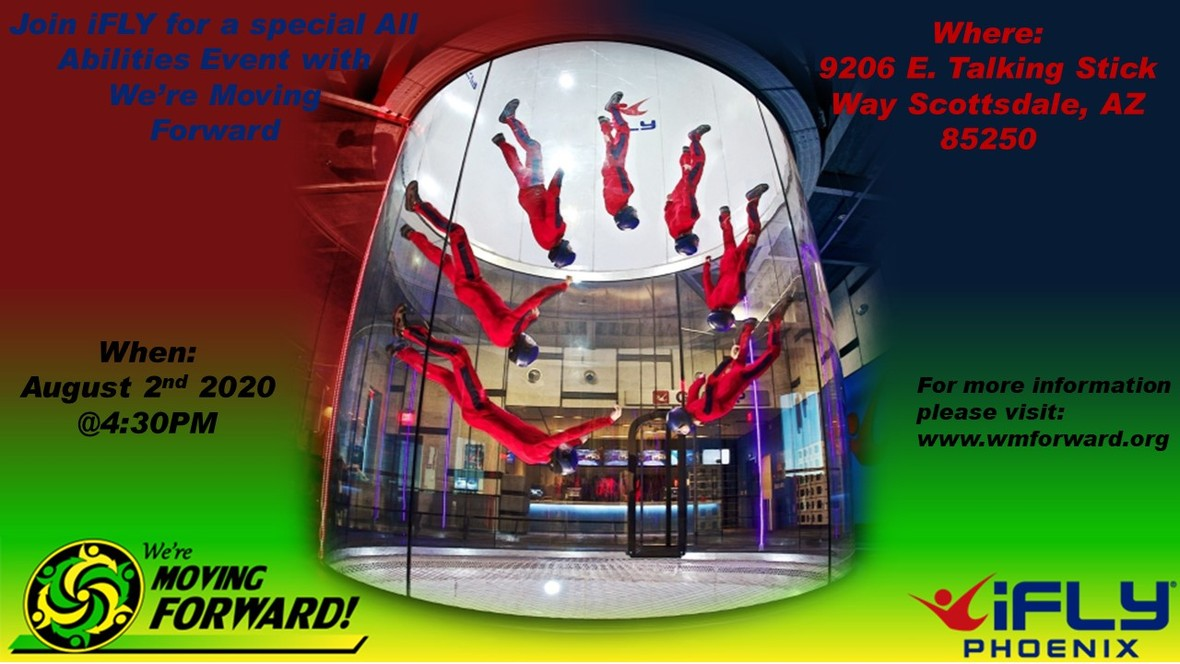/* styles */ FREE for survivors and immediate family/caregivers. \$44 Everyone Else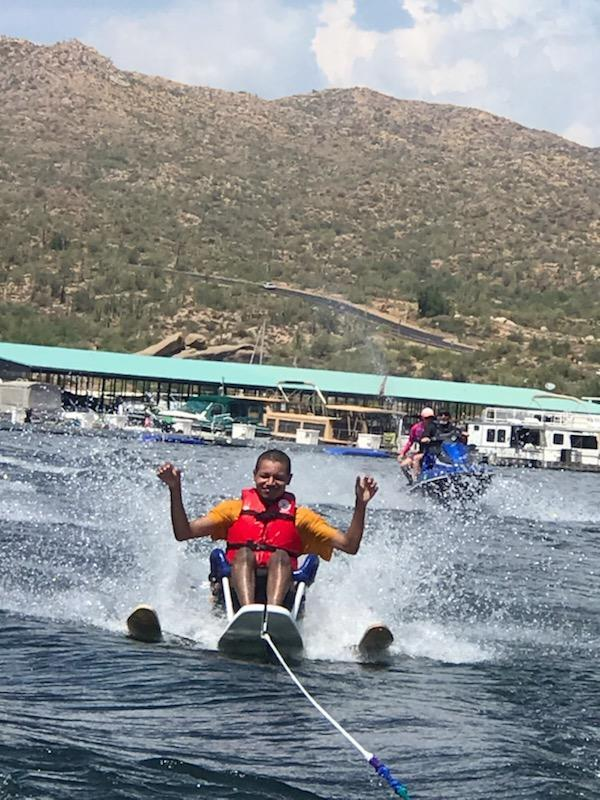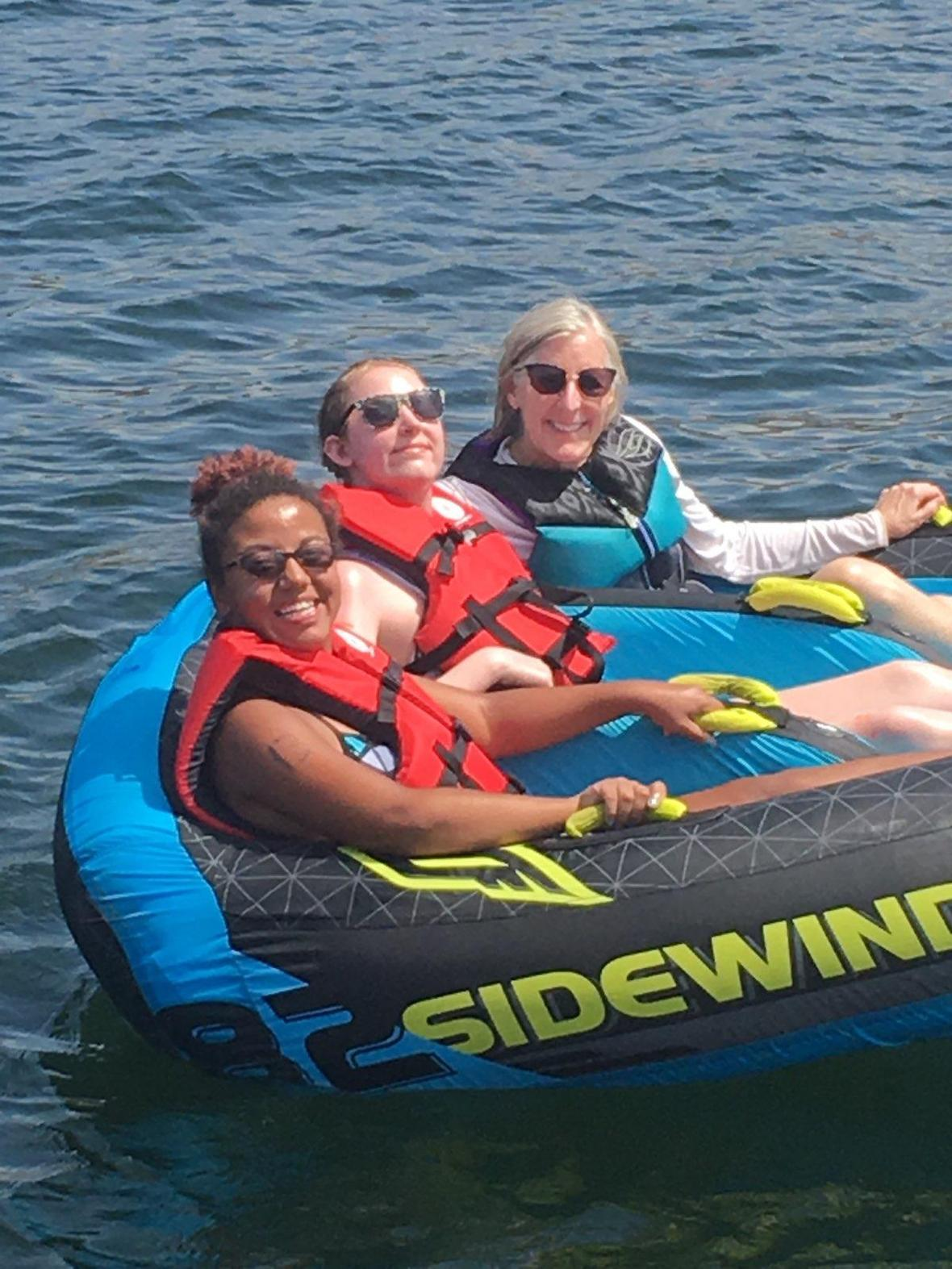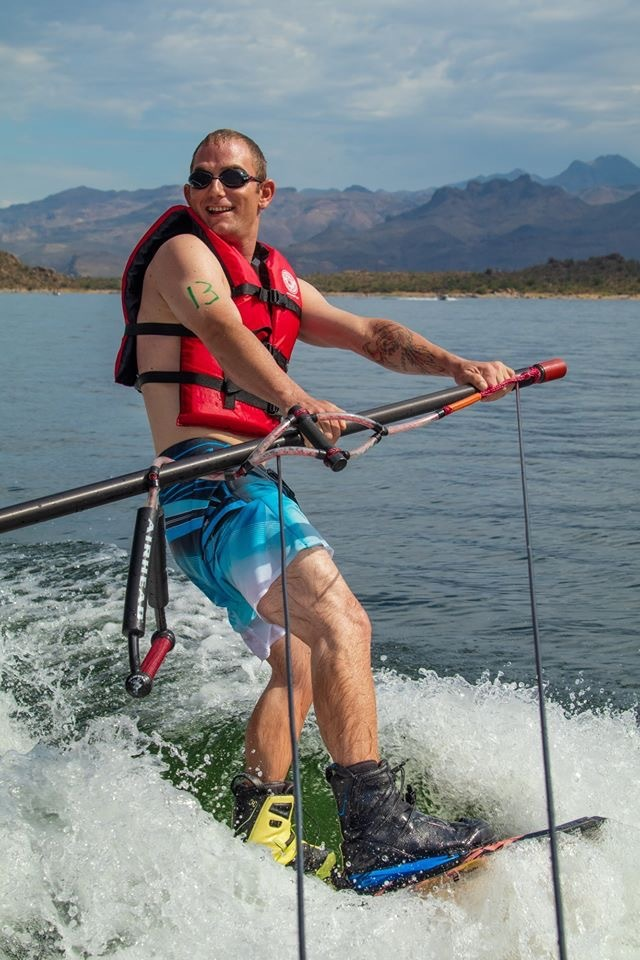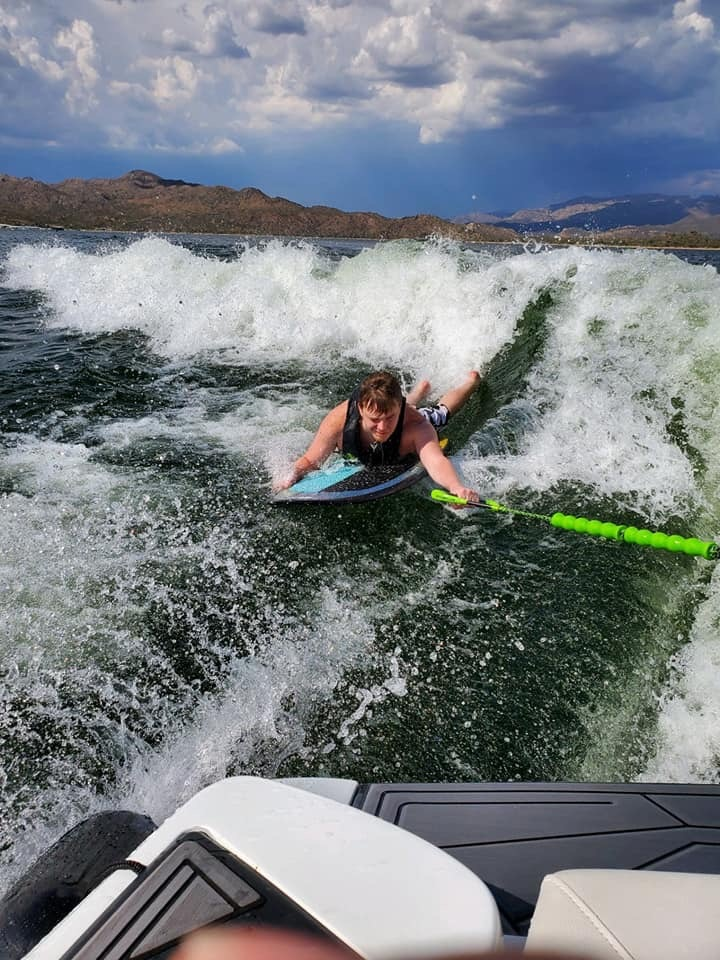/* styles */ September 11th and 12th Adaptive Watersports Experience Days thru Arizona Adaptive Watersports Bartlett Lake Marina Carefree FREE for survivors ages 18+ (if younger, please contact us at info@wmforward.org prior to registering.) (1) Day \$25 with WMF paying the remaining cost (2) Days \$110 (\$25 one day / \$85 2nd day) Spaces fill up fast! Get registered ASAP! DEADLINE to register is 8/27/20.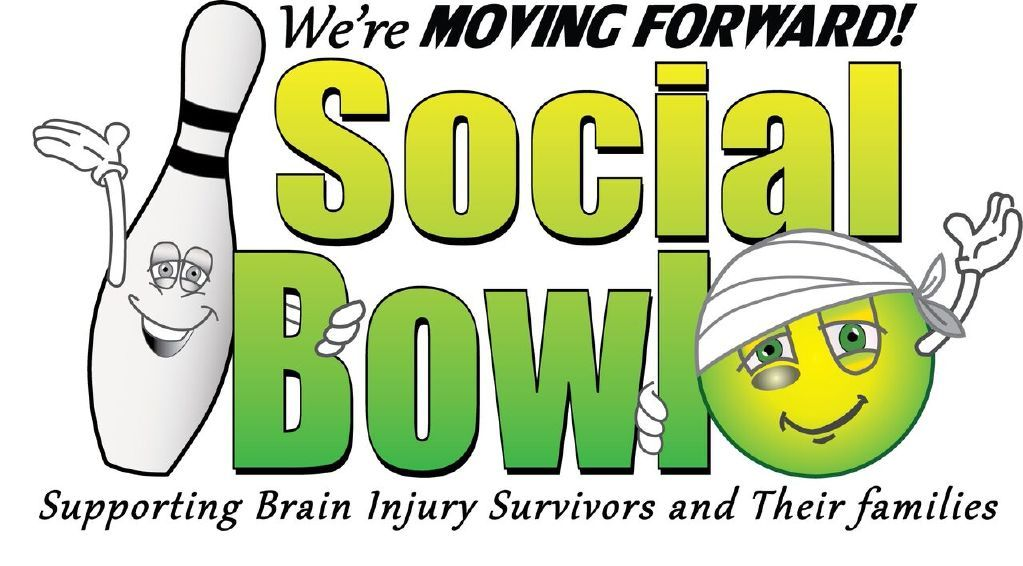September 27th

Social Bowl 2020 will take place at Fat Cats in Gilbert again this year.

Participant registration and sponsorship opportunities will be available soon.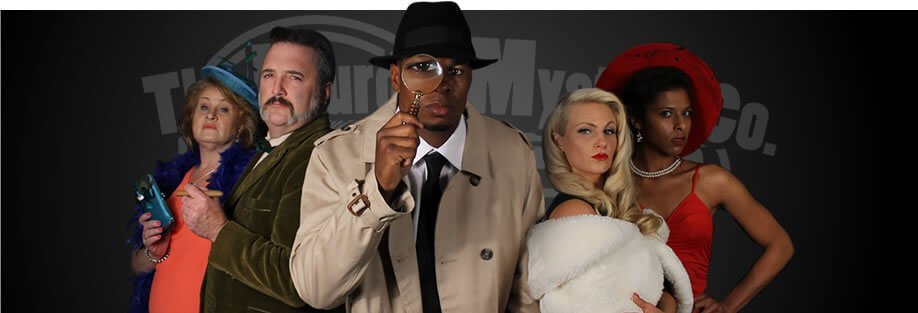October 24th

Murder Mystery Dinner

Ability360

Phoenix

Ages 18+

FREE for survivors and immediate family/survivors

\$15 Everyone elseWe thank Everlasting Community Services for helping to sponsor this activity.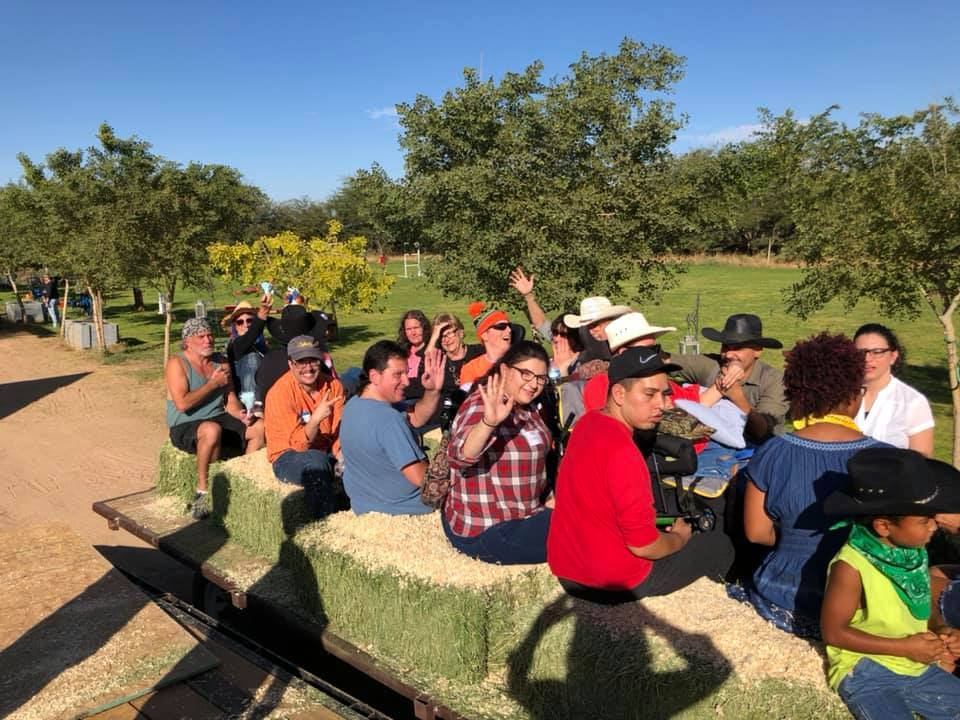/* styles */ November 7th Annual Day in the Country Everyone is welcome AND we need volunteers! Maricopa FREE for survivors and immediate family/caregivers \$5 for ages 4 - 13 \$10 for ages 13 and up DEADLINE to register is 10/29/20December 5th

Annual Christmas Dinner & Dance

Location and Theme TBD

SAVE THE DATE!

 table div table+table+table+table+table+table+table+table+table+table+table+table+table+table+table+table+table+table+table+table+table+table+table+table+table+table+table+table+table+table+table+table+table+table+table+table+table+table+table+table+table+table+table+table+table+table+table div table{width:100%;padding:0}table div table+table+table+table+table+table+table+table+table+table+table+table+table+table+table+table+table+table+table+table+table+table+table+table+table+table+table+table+table+table+table+table+table+table+table+table+table+table+table+table+table+table+table+table+table+table+table div table img{width:96.23%;padding:0;float:none}table div table+table+table+table+table+table+table+table+table+table+table+table+table+table+table+table+table+table+table+table+table+table+table+table+table+table+table+table+table+table+table+table+table+table+table+table+table+table+table+table+table+table+table+table+table+table+table div table td{width:100%;padding:0 1.88% 18px}/* styles */# COMMUNITY CORNER

 table div table+table+table+table+table+table+table+table+table+table+table+table+table+table+table+table+table+table+table+table+table+table+table+table+table+table+table+table+table+table+table+table+table+table+table+table+table+table+table+table+table+table+table+table+table+table+table+table+table div table{width:100%;padding:0}table div table+table+table+table+table+table+table+table+table+table+table+table+table+table+table+table+table+table+table+table+table+table+table+table+table+table+table+table+table+table+table+table+table+table+table+table+table+table+table+table+table+table+table+table+table+table+table+table+table div table img{width:96.23%;padding:0;float:none}table div table+table+table+table+table+table+table+table+table+table+table+table+table+table+table+table+table+table+table+table+table+table+table+table+table+table+table+table+table+table+table+table+table+table+table+table+table+table+table+table+table+table+table+table+table+table+table+table+table div table td{width:100%;padding:0 1.88% 18px}/* styles */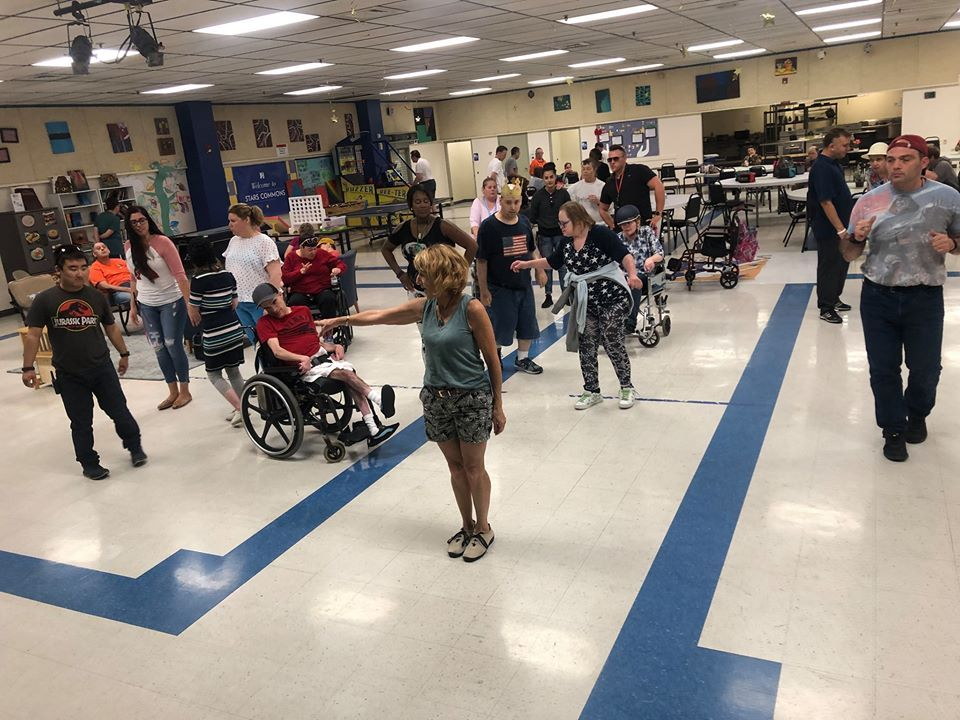NEW Adaptive Dance And Movement Class

Vickie Aigner presents her Alive, Fit & Free Adaptive Dance and Movement class at Naam Arizona

At Alive, Fit & Free, we are passionate about bringing wellness to every person and we specialize in adapting fitness and health classes to fit your abilities.
We believe health and wellness are the cornerstones of a high quality of life. Everyone deserves a high quality of life, regardless of their ability. We created Alive, Fit & Free to make this a reality.
FOR EVERY ABILITY.

Alive, Fit & Free was born out of a passion to bring a higher quality of life to clients with unique abilities who have not been catered to by the traditional health and wellness market.

What is Adaptive Dance and Movement?
Adaptive dance and movement is a unique system of Naam Yoga & Dance Movement that is adaptable to all levels and abilities, whether clients are confined to a chair or have the freedom to use their whole bodies.

It energizes emotionally, mentally, energetically and physically from the inside out with its unique energy and movement patterns.

This, in turn, relieves stress, depression and anxiety, builds new neuro-pathways in the brain, helps to develop neuroplasticity, increases balance and coordination, enhances flexibility, opens up our energetic pathways, increases cardio vascular output, and stimulates muscular strength and endurance – all while having fun and allowing a safe space for all to open wide and explore their inner beings.

Every 2nd & 4th Wednesday

Begins:
Wednesday, January 8
3:00 - 4:15
\$12 per class

NAAM YOGA ARIZONA
5050 E UNIVERSITY DR SUITE 114
MESA, AZ 85205
480-458-7497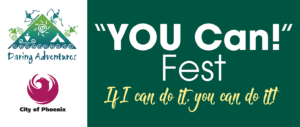/* styles */ February 1st WMF will be at Daring Adventures - "You Can! Fest" - "If I Can Do It - You Can Do It" "Click Here" for details. Stop by and visit us!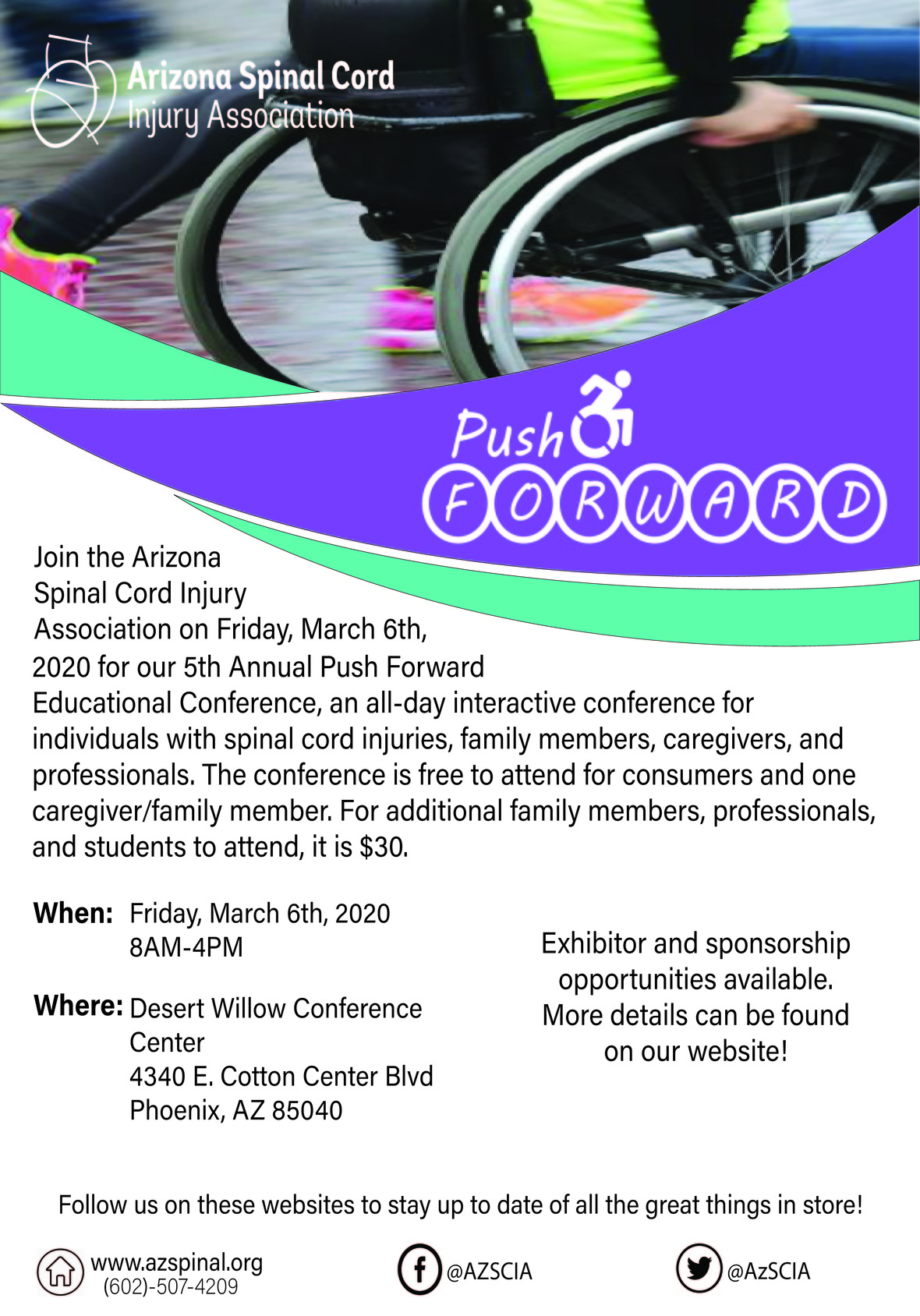https://www.azspinal.org/conferences.html
 /* styles */ WMF will be at the Push Forward Conference and the Brain Injury Survivor & Caregiver Expo - please make sure to stop by and say hello!
 table div table+table+table+table+table+table+table+table+table+table+table+table+table+table+table+table+table+table+table+table+table+table+table+table+table+table+table+table+table+table+table+table+table+table+table+table+table+table+table+table+table+table+table+table+table+table+table+table+table+table+table+table+table+table+table div table td,table.module-54{width:100%;padding:0}table div table+table+table+table+table+table+table+table+table+table+table+table+table+table+table+table+table+table+table+table+table+table+table+table+table+table+table+table+table+table+table+table+table+table+table+table+table+table+table+table+table+table+table+table+table+table+table+table+table+table+table+table+table+table+table div table{width:100%;float:none;margin-left:auto;margin-right:auto;padding:0}table div table+table+table+table+table+table+table+table+table+table+table+table+table+table+table+table+table+table+table+table+table+table+table+table+table+table+table+table+table+table+table+table+table+table+table+table+table+table+table+table+table+table+table+table+table+table+table+table+table+table+table+table+table+table+table div table a{border:0 none;text-decoration:none}table div table+table+table+table+table+table+table+table+table+table+table+table+table+table+table+table+table+table+table+table+table+table+table+table+table+table+table+table+table+table+table+table+table+table+table+table+table+table+table+table+table+table+table+table+table+table+table+table+table+table+table+table+table+table+table div table img{width:100%!important;border:0 none;text-decoration:none}/* styles */
 table div table+table+table+table+table+table+table+table+table+table+table+table+table+table+table+table+table+table+table+table+table+table+table+table+table+table+table+table+table+table+table+table+table+table+table+table+table+table+table+table+table+table+table+table+table+table+table+table+table+table+table+table+table+table+table+table div table,table.module-55{width:71.7%;float:left;padding:0}table div table+table+table+table+table+table+table+table+table+table+table+table+table+table+table+table+table+table+table+table+table+table+table+table+table+table+table+table+table+table+table+table+table+table+table+table+table+table+table+table+table+table+table+table+table+table+table+table+table+table+table+table+table+table+table+table div table a{border:0 none;text-decoration:none}table div table+table+table+table+table+table+table+table+table+table+table+table+table+table+table+table+table+table+table+table+table+table+table+table+table+table+table+table+table+table+table+table+table+table+table+table+table+table+table+table+table+table+table+table+table+table+table+table+table+table+table+table+table+table+table+table div table img{width:100%!important;border:0 none;text-decoration:none}table div table+table+table+table+table+table+table+table+table+table+table+table+table+table+table+table+table+table+table+table+table+table+table+table+table+table+table+table+table+table+table+table+table+table+table+table+table+table+table+table+table+table+table+table+table+table+table+table+table+table+table+table+table+table+table+table div table td{width:100%;padding:0 20px 20px 0}/* styles */ Camp With A Ramp 2020 (4th year) is on for July 30th - Aug 2nd. Start saving your money now people!! Only \$20 a month and BAM you’re there! 3 days and nights FULL of activities, transportation, meals, lodging, caregiver and volunteer support, out of Phoenix heat.

AUGUST 6 – 9, 2020

Payson, Arizona

Camp “Can Do” is a four-day camp for adult survivors of brain injury. A signature program of the Brain Injury Alliance of Arizona, for over 10 years Camp Can Do has helped survivors of serious brain injury have interactions with nature, form lifelong friendships, and enjoy independence from caregivers. Camp is a life changing experience for many. Physical and occupational therapy students serve as volunteers at camp and are able to gain real world experience assisting campers and facilitating recreation and social activities appropriate for those with cognitive challenges.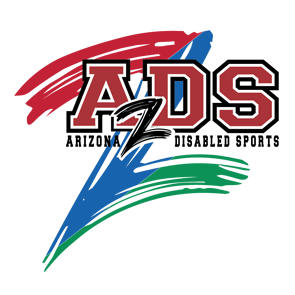https://www.arizonadisabledsports.com//* styles */ The 2020 SkiAble Adaptive Alpine Experience presented by the Dralla Foundation will take place February 2 - 4, 2020 at the Arizona Snowbowl in Flagstaff, Arizona. This is an opportunity for individuals with a physical disability or visual impairment to experience an unforgettable day on the slopes! No prior skiing experience is necessary, as lessons can accommodate beginner, intermediate and experienced skiers. Click on the AZDS website info above.
 table div table+table+table+table+table+table+table+table+table+table+table+table+table+table+table+table+table+table+table+table+table+table+table+table+table+table+table+table+table+table+table+table+table+table+table+table+table+table+table+table+table+table+table+table+table+table+table+table+table+table+table+table+table+table+table+table+table+table+table+table div table{width:100%;padding:0}table div table+table+table+table+table+table+table+table+table+table+table+table+table+table+table+table+table+table+table+table+table+table+table+table+table+table+table+table+table+table+table+table+table+table+table+table+table+table+table+table+table+table+table+table+table+table+table+table+table+table+table+table+table+table+table+table+table+table+table+table div table img{width:96.23%;padding:0;float:none}table div table+table+table+table+table+table+table+table+table+table+table+table+table+table+table+table+table+table+table+table+table+table+table+table+table+table+table+table+table+table+table+table+table+table+table+table+table+table+table+table+table+table+table+table+table+table+table+table+table+table+table+table+table+table+table+table+table+table+table+table div table td{width:100%;padding:0 1.88% 18px}/* styles */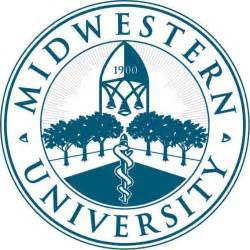If you are a survivor that lives in the west valley or would be willing to travel that direction, there is an opportunity to help Midwestern University students learn from working with you.

Email info@wmforward.org and you will be provided with the contact information.

 table div table+table+table+table+table+table+table+table+table+table+table+table+table+table+table+table+table+table+table+table+table+table+table+table+table+table+table+table+table+table+table+table+table+table+table+table+table+table+table+table+table+table+table+table+table+table+table+table+table+table+table+table+table+table+table+table+table+table+table+table+table+table div table{width:100%;padding:0}table div table+table+table+table+table+table+table+table+table+table+table+table+table+table+table+table+table+table+table+table+table+table+table+table+table+table+table+table+table+table+table+table+table+table+table+table+table+table+table+table+table+table+table+table+table+table+table+table+table+table+table+table+table+table+table+table+table+table+table+table+table+table div table img{width:96.23%;padding:0;float:none}table div table+table+table+table+table+table+table+table+table+table+table+table+table+table+table+table+table+table+table+table+table+table+table+table+table+table+table+table+table+table+table+table+table+table+table+table+table+table+table+table+table+table+table+table+table+table+table+table+table+table+table+table+table+table+table+table+table+table+table+table+table+table div table td{width:100%;padding:0 1.88% 18px}/* styles */# Treasurer's Note

 /* styles */ We made it through our 5th year as a non-profit due to your generosity and support!If for any reason you misplaced a WMF donation receipt or did not receive one (including in-kind services), please contact Tara Pepiton, (602) 909-7330 or tpepiton@wmforward.org no later than 1/8/20.

 table div table+table+table+table+table+table+table+table+table+table+table+table+table+table+table+table+table+table+table+table+table+table+table+table+table+table+table+table+table+table+table+table+table+table+table+table+table+table+table+table+table+table+table+table+table+table+table+table+table+table+table+table+table+table+table+table+table+table+table+table+table+table+table+table+table+table div table{width:100%;padding:0}table div table+table+table+table+table+table+table+table+table+table+table+table+table+table+table+table+table+table+table+table+table+table+table+table+table+table+table+table+table+table+table+table+table+table+table+table+table+table+table+table+table+table+table+table+table+table+table+table+table+table+table+table+table+table+table+table+table+table+table+table+table+table+table+table+table+table div table img{width:96.23%;padding:0;float:none}table div table+table+table+table+table+table+table+table+table+table+table+table+table+table+table+table+table+table+table+table+table+table+table+table+table+table+table+table+table+table+table+table+table+table+table+table+table+table+table+table+table+table+table+table+table+table+table+table+table+table+table+table+table+table+table+table+table+table+table+table+table+table+table+table+table+table div table td{width:100%;padding:0 1.88% 18px}/* styles */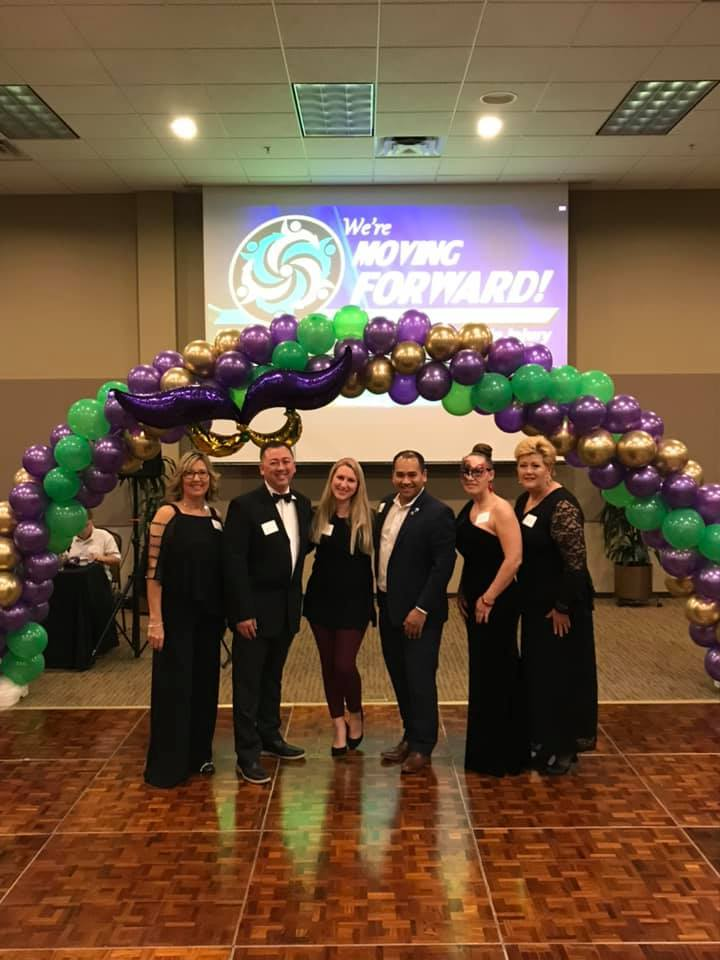WMF BOD Ronda Alcorn, Justin Larson, Lauren Bostick, Chey Tor, Michelle Lauer and Tara Pepiton
 /* styles */
 /* styles */ We're MOVING FORWARD! Contacts Ronda Alcorn, Founder/President (480) 209-7663 ralcorn@wmforward.org Tara Pepiton, Founder/Treasurer (602) 909-7330 tpepiton@wmforward.org
 Like   Tweet